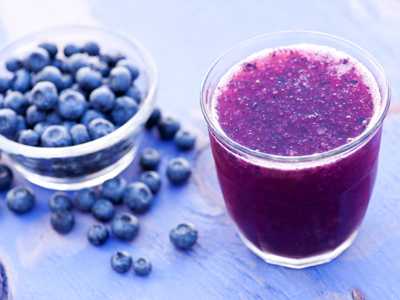How do we measure liquids?

# Measurements - Gallons, Quarts and Pints

This Math quiz is called 'Measurements - Gallons, Quarts and Pints' and it has been written by teachers to help you if you are studying the subject at middle school. Playing educational quizzes is a fabulous way to learn if you are in the 6th, 7th or 8th grade - aged 11 to 14.

It costs only \$12.50 per month to play this quiz and over 3,500 others that help you with your school work. You can subscribe on the page at Join Us

Different objects have different ways of being measured. For this quiz, we will be measuring volume of liquids. A gallon is a unit measure of volume. For example, if we want to buy milk, we will buy the milk in volume. We then need to decide how much volume we wish to buy it in. We can buy it in a gallon, in a quart or in a pint.

A gallon can be broken down into smaller volumes of measurement that include quarts and pints.

There are 4 quarts in one gallon and there are 8 pints in one gallon. So:

4 quarts = 1 gallon
8 pints = 1 gallon

There are then 2 pints in every quart. So if there are 2 pints in every quart, how many pints are in a gallon? We simply multiply 4 quarts by 2 pints or 4 x 2 = 8. This is how we know we have 8 pints in a gallon.

If you want to know how many pints are in 4 gallons, you would simply multiply 4 (gallons) by 8 (pints) or 4 x 8 = 32 so there are 32 pints in 4 gallons.

If you want to know how many quarts are in 4 gallons, you would simply multiply 4 (gallons) by 4 quarts or 4 x 4 = 16 so there are 16 quarts in 4 gallons.

Now, if you know the number of pints you have but you need to convert that into gallons, you would then divide. For example, if you have 144 pints and there are 8 pints in a gallon, you would then divide 144 by 8 or 144 ÷ 8 = 18 so your 144 pints equals 18 gallons.

If you have 144 quarts and you need to convert to gallons, you would divide 144 quarts by 4 because there are 4 quarts in one gallon. Therefore, 144 ÷ 4 = 36 so your 144 quarts equals 36 gallons.

1.
28 gallons = _____ quarts
7
112
224
56
There are 4 quarts in 1 gallon so we need to multiply 28 gallons by 4 quarts or 28 x 4 = 112. 28 gallons = 112 quarts. Answer (b) is the correct answer
2.
8 quarts = ____ pints
12
32
16
24
There are 2 pints in 1 quart so we need to multiply 8 quarts by 2 pints or 8 x 2 = 16. 8 quarts = 16 pints. Answer (c) is the correct answer
3.
132 pints = ____ quarts
246
66
33
22
There are 2 pints in 1 quart so we need to divide 132 pints by 2 pints or 132 ÷ 2 = 66. This tells us that 132 pints = 66 quarts. Answer (b) is the correct answer
4.
100 gallons = ____ quarts = ____ pints
200 quarts = 400 pints
400 quarts = 800 pints
800 quarts = 1600 pints
1600 quarts = 3200 pints
There are 4 quarts in 1 gallon so we need to multiply 100 gallons by 4 quarts or 100 x 4 = 400. 100 gallons = 400 quarts. There are 2 pints in 1 quart so we need to multiply 400 quarts by 2 pints or 400 x 2 = 800. 400 quarts = 800 pints. So 100 gallons = 400 quarts = 800 pints. Answer (b) is the correct answer
5.
200 gallons = ____ pints
400
800
1200
1600
There are 8 pints in 1 gallon so we need to multiply 200 gallons by 8 pints or 200 x 8 = 1600. 200 gallons = 1600 pints. Answer (d) is the correct answer
6.
64 pints = ____ gallons
8
32
24
10 gallons 4 pints or 10 gallons 2 quarts
There are 8 pints in 1 gallon so we need to divide 64 pints by 8 pints or 64 ÷ 8 = 8. This tells us that 64 pints = 8 gallons. Answer (a) is the correct answer
7.
19 gallons = ____ pints
76
38
114
152
There are 8 pints in 1 gallon so we need to multiply 19 gallons by 8 pints or 19 x 8 = 152. 19 gallons = 152 pints. Answer (d) is the correct answer
8.
860 quarts = ____ gallons
215
1720
108
430
There are 4 quarts in 1 gallon so we need to divide 860 quarts by 4 quarts or 860 ÷ 4 = 215. This tells us that 860 quarts = 215 gallons. Answer (a) is the correct answer
9.
15 quarts =____ pints
120
60
30
90
There are 2 pints in 1 quart so we need to multiply 15 quarts by 2 pints or 15 x 2 = 30. 15 quarts = 30 pints. Answer (c) is the correct answer
10.
47 gallons = _____ quarts
94
12
376
188
There are 4 quarts in 1 gallon so we need to multiply 47 gallons by 4 quarts or 47 x 4 = 188. 47 gallons = 188 quarts. Answer (d) is the correct answer
Author:  Christine G. Broome